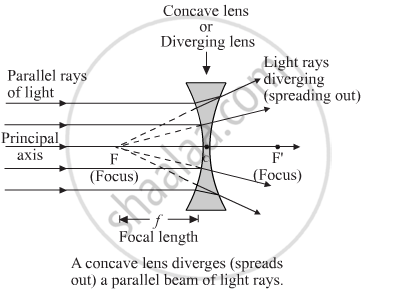Share

# With the Help of a Labelled Diagram, Explain How a Concave Lens Diverges a Beam of Parallel Light Rays. Mark the Principal Axis, Optical Centre, Principal Focus and Focal Length of the Concave Lens on the Diagram. - CBSE Class 10 - Science

ConceptRepresentation of Images Formed by Spherical Mirrors Using Ray Diagrams - Concave Mirror

#### Question

With the help of a labelled diagram, explain how a concave lens diverges a beam of parallel light rays. Mark the principal axis, optical centre, principal focus and focal length of the concave lens on the diagram.

#### Solution

When a parallel beam of light rays falls on a concave lens, the rays spread out (or diverge) after passing through the lens (figure). Since the refracted rays are diverging away from one another, they do not actually meet at a point. The diverging rays, when produced backwards appear to meet at a point F called the principal focus, on the left side of the lens.Is there an error in this question or solution?

#### APPEARS IN

Solution With the Help of a Labelled Diagram, Explain How a Concave Lens Diverges a Beam of Parallel Light Rays. Mark the Principal Axis, Optical Centre, Principal Focus and Focal Length of the Concave Lens on the Diagram. Concept: Representation of Images Formed by Spherical Mirrors Using Ray Diagrams - Concave Mirror.
S